# 2. 实验

## 2.2 测量土壤电特性

### 2.2.1 土壤电阻率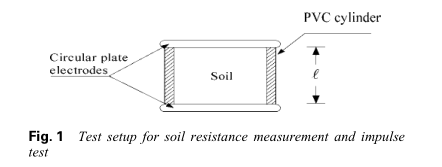$\rho _ { g } = \frac { R a } { \ell } = \frac { 1 } { \sigma _ { g } } ( \Omega m ) \tag{1}$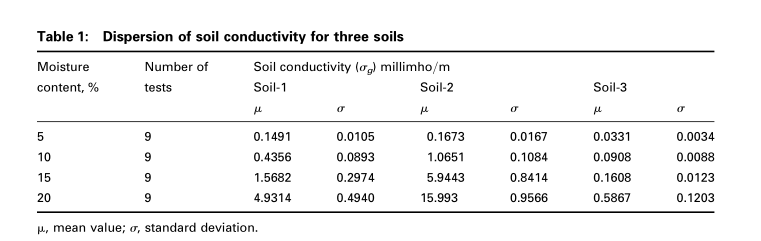### 2.2.2 土壤介电常数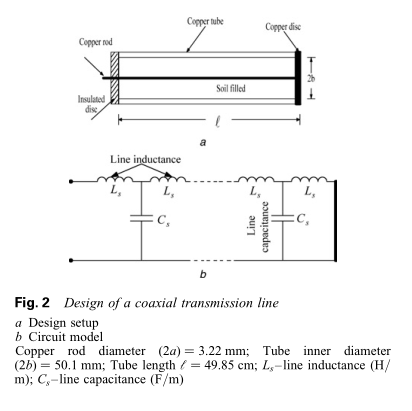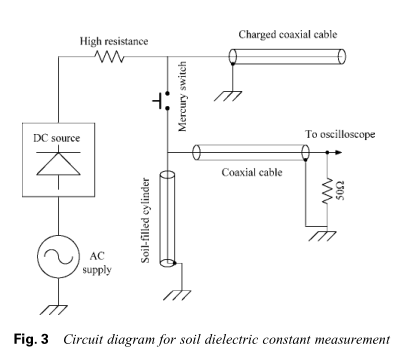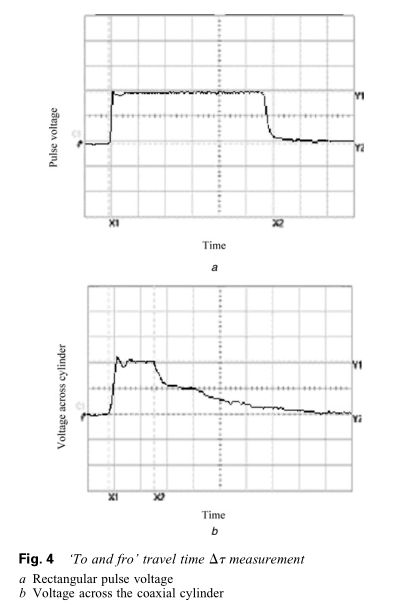$v _ { c } = \frac { 2 \ell } { \Delta \tau } = \frac { 1 } { \sqrt { L _ { s } C _ { s } } } = \frac { 1 } { \sqrt { L _ { s } ( C _ { 0 } k _ { g } ) } } = \frac { c } { \sqrt { k _ { g } } } \tag{2}$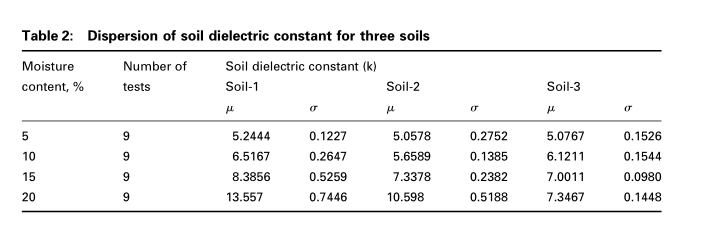### 2.2.3 均匀电场下的土壤临界电场$E_c$

$E _ { c } = \frac { V _ { \text { withstand } } } { \ell } ( kV / m ) \tag{4}$

# 3. 结论

## 3.1 波形对土壤临界电场$E_c$的意义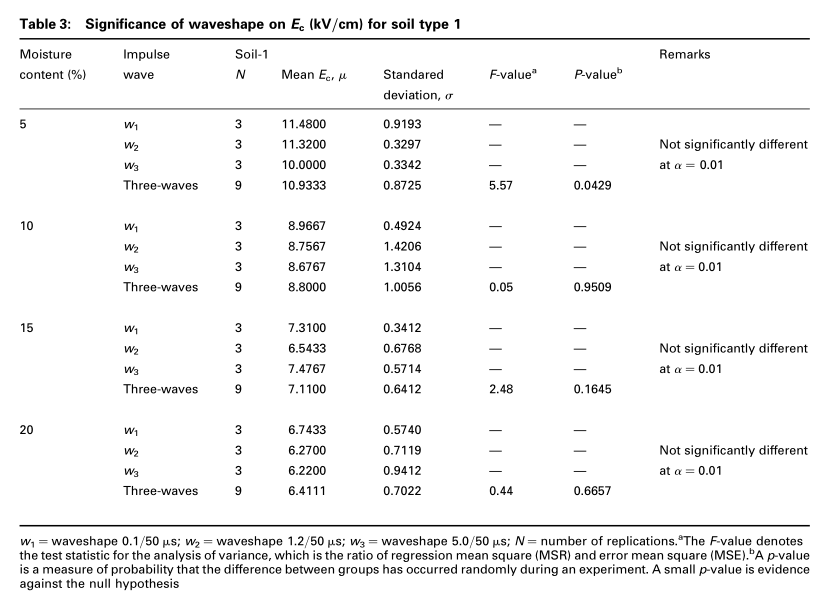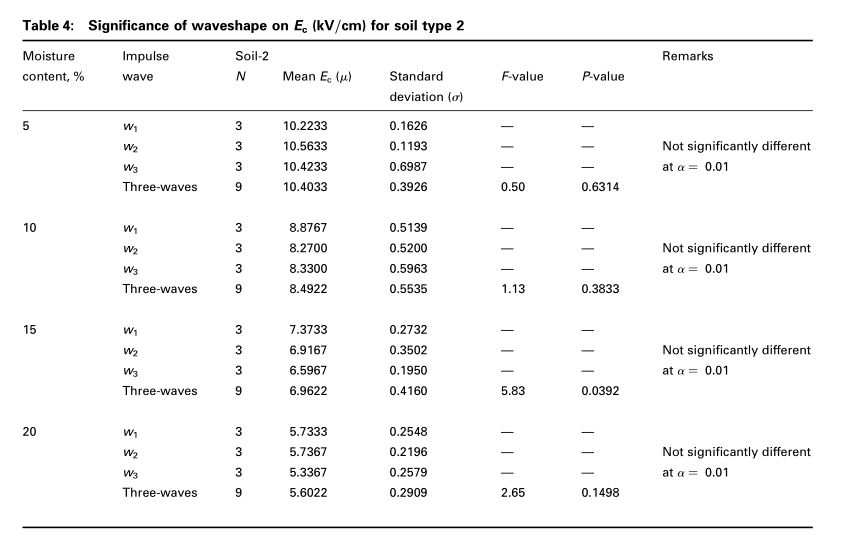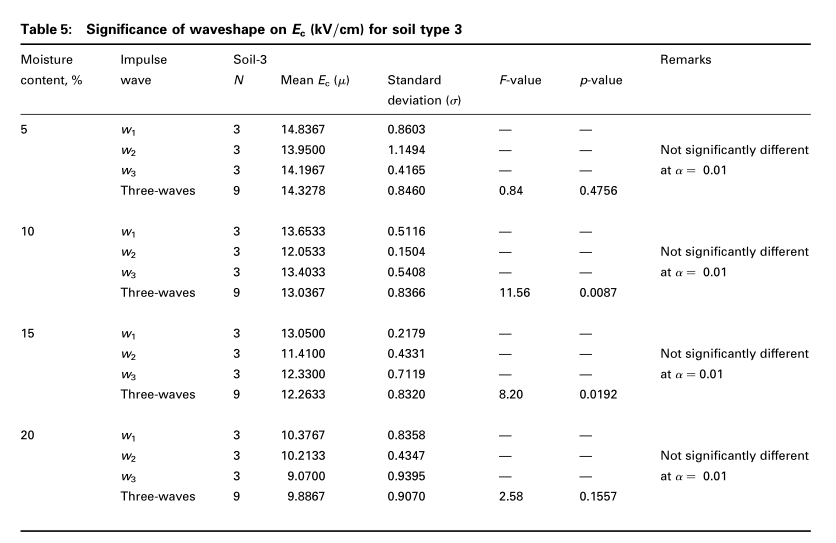## 3.3 土壤分解的物理解释

$E _ { 1 } = \frac { \varepsilon _ { 2 } } { \varepsilon _ { 1 } } E _ { 2 } \tag{5}$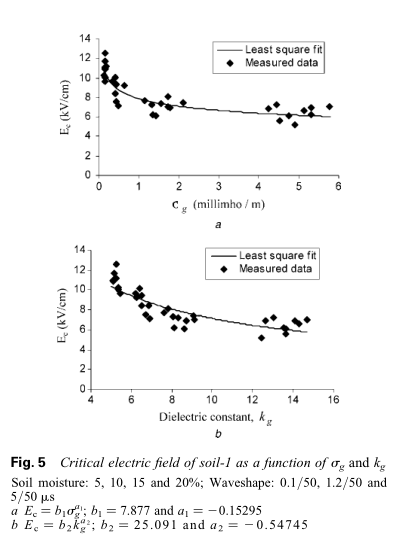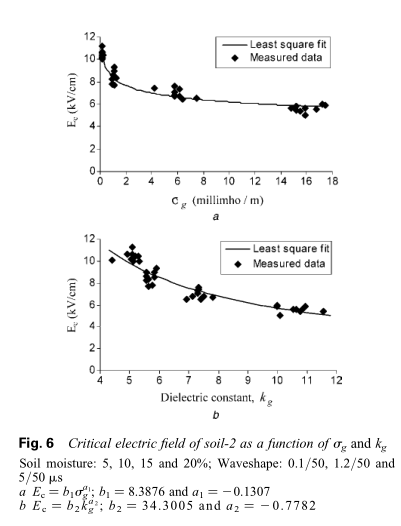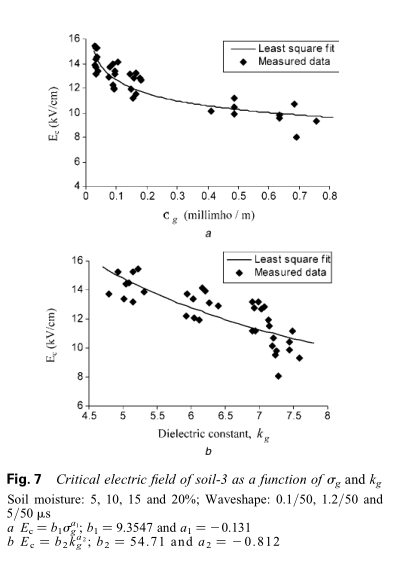## 3.4 提出$E_c$模型

$\operatorname { log } E _ { c } = \beta _ { 0 } + \beta _ { 1 } * \operatorname { log } k _ { g } + \beta _ { 2 } * \operatorname { log } \sigma _ { g } + \varepsilon _ { i } \tag{6}$

$E _ { c } = 8.6083 * ( k _ { g } ) ^ { - 0.0103 } * ( \sigma _ { g } ) ^ { - 0.1526 } \tag{7}$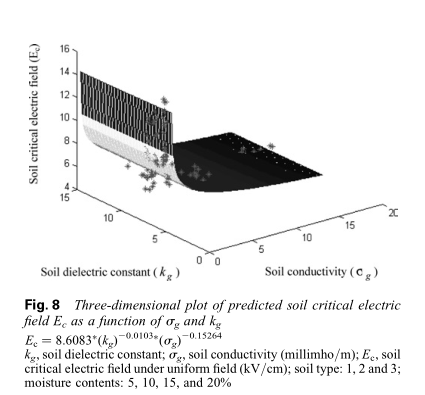## 3.5 土壤样品的多重分解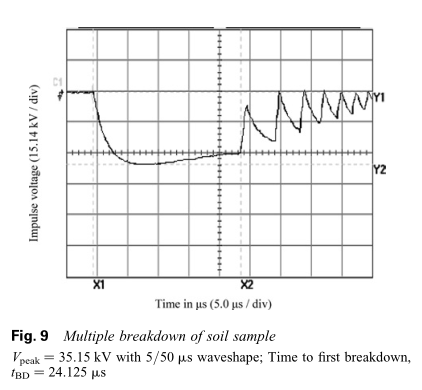# 4. 结论

1.土壤的介电常数k g可以通过波传播技术使用充满土壤的同轴传输线来测量，这消除了极化效应。 这是一种创新方法。

2.施加的脉冲电压的波形对土壤临界电场$E_c$没有明显影响。

3.土壤临界电场的新的广义方程$E _ { c } = 8.6083 ^ { * } ( k _ { g } ) ^ { - 0.0103 * } ( \sigma _ { g } ) ^ { - 0.1526 } ( kV / cm )$，是在考虑大量土壤参数的广泛实验室测试基础上提出的，适用于任何土壤条件。

4.可以通过将$E_c$模型纳入接地系统动态模型中来估算脉冲阻抗，这是合理而准确的。